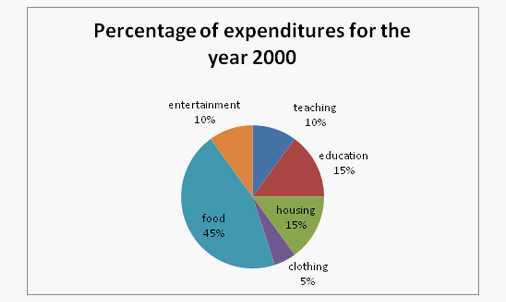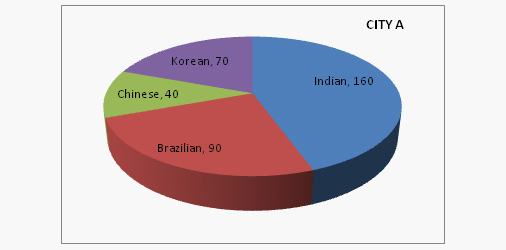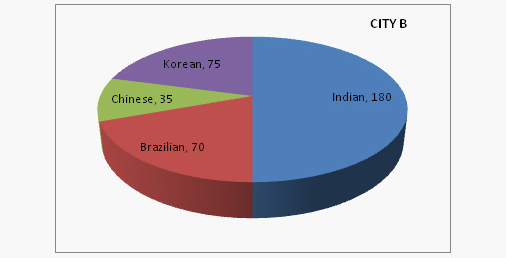# Pie Chart Practice Questions: Level 01

DIRECTIONS for questions 1-5: The pie chart given below shows the spending of a family on different articles for the year 2000. Analyse the pie chart and answer the questions which follow.1. If the total amount that was spent in the year 2000 was 50000, the amount that was spent on food was
A. Rs.225000
B. Rs.22500
C. Rs.24000
D. Rs.21400
2. If the total amount that was spent in the year2000 was Rs. 48000, the amount that was spent on housing and clothing combined was
A. Rs.9600
B. Rs.8000
C. Rs.10000
D. Rs.12000
3. The ratio of the amount spent on teaching and education to clothing and food was
A. 3:2
B. 2:3
C. 1:5
D. 1:2
4. According to the graph, the maximum amount that was spent was on which item (if the expenditure that is given to be Rs. 58256)?
A. Food
B. Housing
C. Clothing
D. Others
5. If the amount spent during the year 2000 was Rs. 62000, the amount spent on clothing during the year was
A. Rs. 1500
B. Rs. 15000
C. Rs. 3100
D. Rs. 3200
The following pie charts give the 'exact values' of people of different counties settled in City A and City B in the form of two pie charts.1. Indians which were in the city A form what percent of the total residents in the same city?
A. More than 50%
B. 25%
C. Less than 50%
D. Unknown
2. Indians in city B are what percent of the total persons?
A. 40%
B. 50%
C. 12.5%
3. Koreans of city A are what percent of the Koreans given in the city B?
A. 93
B. 97
C. 96
D. 90
4. Total Brazilians of both the cities form what percent of the total Indians given in both the charts?
A. 22.22%
B. 28.57%
C. 47%
D. 41.61%
5. Koreans of city A form what percent of Chinese of city B?
A. 200%
B. 100%
C. 50%
D. 150%